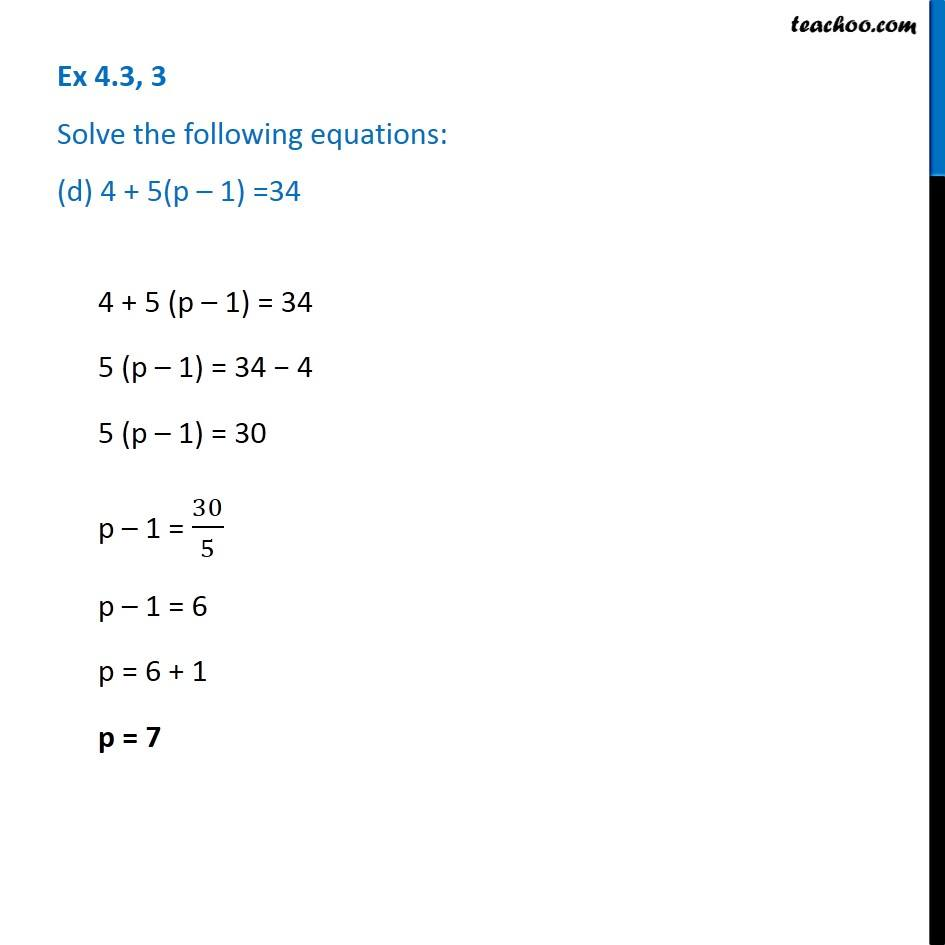1. Chapter 4 Class 7 Simple Equations
2. Serial order wise
3. Ex 4.3

Transcript

Ex 4.3, 3 Solve the following equations: (d) 4 + 5(p – 1) =344 + 5 (p – 1) = 34 5 (p – 1) = 34 − 4 5 (p – 1) = 30 p – 1 = 30/5 p – 1 = 6 p = 6 + 1 p = 7

Ex 4.3

Chapter 4 Class 7 Simple Equations
Serial order wise